Next: About this document ... Up: lab_template Previous: lab_template

Subsections

# Implicit Differentiation

### Implicit Differentiation

The implicitdiff command can be used to find derivatives of implicitly defined functions. Suppose we wanted to use implicit differentiation to find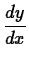for the relationThen we first define our relation and give it a label for later use.
> f:=x^2*y^2+y^3=0;

Note that the expression syntax is used as the equal sign is entered as part of the command. You cannot use function notation! The syntax of the implicitdiff command is shown by the following example.
> implicitdiff(f,y,x);


The result of the command is the derivative,. The first argument is the relation that you want to differentiate implicitly. The second argument to the implicitdiff command is where you tell Maple what the dependent variable is. The remaining argument is to specifying the derivative you want.

To compute numerical values of derivatives obtained by implicit differentiation, you have to use the subs command. For example, to find the value ofat the pointyou could use the following command.

> subs([x=1,y=-1],implicitdiff(f,y,x));


## Plotting and solving implicitly

First of all if you are going to plot implicitly you need to load the plots package.
> with(plots):

The implicitplot, solve, and fsolve commands have syntax similar to what you are familiar with. Just remember that you are dealing with two variablesand
. When graphing the two equations in implicitplot you must specify the domain AND range.
> f := x^2*y^2+y^3 = 0;g := x^2*sin(y) = 1;
> implicitplot([f,g],x=-10..10,y=-10..0);

You can add options to the plot command to make the picture more clear. Execute the following commands to see the improvements.
> implicitplot([f,g],x=-10..10,y=-10..0,numpoints=10000);
> implicitplot([f,g],x=-10..10,y=-10..0,numpoints=10000,color=[Aqua'',
Magenta'']);
> implicitplot([f,g],x=-10..10,y=-10..0,numpoints=10000,color=[Aqua'',
Magenta''],scaling=constrained);

To find where the graphs intersect you can use the solve or fsolve command. Since you need to solve two equations with two unknowns you need to solve simultaneously. Simply use curly brackets in the commands to do this.
> a := fsolve({f, g}, {x = -5 .. 0, y = -5 .. 0});
> b := fsolve({f, g}, {x = -5 .. 0, y = -5 .. 0});
> c := fsolve({f, g}, {x = 0 .. 5, y = -5 .. 0});
> d := fsolve({f, g}, {x = 0 .. 5, y = -10 .. 5});

You could then use these points to find the slopes of either function.Then you could use the points and slope values to find the tangent lines. Pay particular attention to how the output-variable name a is used in the commands!!!!
> afslope := subs(a, implicitdiff(f, y, x));
> agslope := evalf(subs(a, implicitdiff(g, y, x)));
> afline := afslope*(x-a)+a;
> agline := agslope*(x-b)+b;
> implicitplot([g, f, afline, agline], x = -10 .. 10, y = -10 .. 5,
numpoints = 10000, color = ["Aqua", "Magenta", "DarkOliveGreen", "Blue"],
scaling = constrained);


## Exercises

1. Enter the following equation as expression
in Maple:
.
A)
Plot the equation using the domain
and the range
. Looking at the graph, how many points have the x value?
B)
Using the solve command find the y values of the point(s). State your point(s) (x,y) in text.
C)
Find the slope of the tangent at each point in part C) and name them
and2. Using the same expression:
A)
Looking at your graph in exercise 1, is the concavity positive or negative at each point whose x value is.
B)
Find
of each of the points.
3. Using the same expression:
A)
Write the equation of the tangent lines for your two points. Make sure to give them each a name.
B)
Plot the expression and the two tangent lines.Next: About this document ... Up: lab_template Previous: lab_template
Jane E Bouchard
2011-10-19